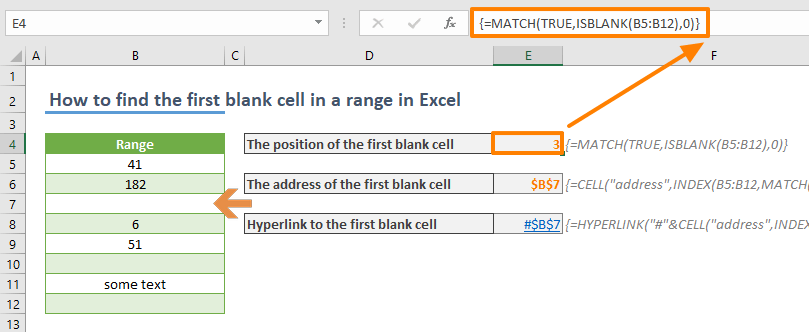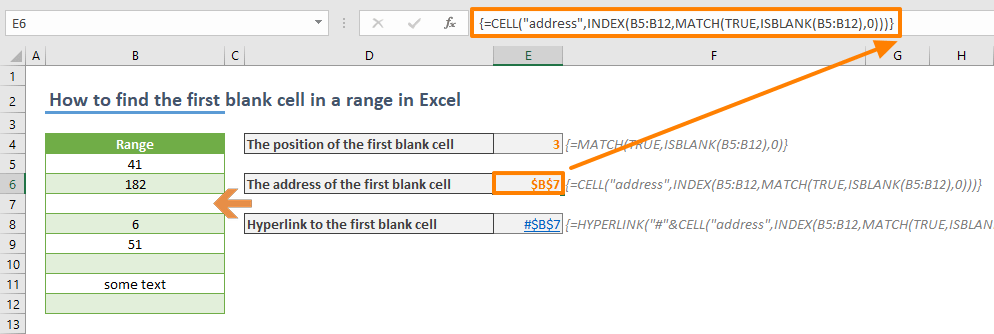Finding the first blank cell in your workbook can become a fairly repetitive task, especially in situations like adding new rows into a large data set. In this article, we are going to show you how to find the first blank cell in a range in Excel, and also show you how to create a hyperlink to cells directly.

## Formula

Finding the position:      {=MATCH(TRUE,ISBLANK(RANGE),0)}

*             range is the reference from the work range

**           {} characters are put by Excel automatically. Do not type them.

## How it works

Excel doesn’t have a built-in formula to find the first blank cell in a range. However, there is the ISBLANK function, which tests a cell, and returns a Boolean value according to its content. The function returns TRUE if cell is blank, FALSE otherwise. Thus, finding the first FALSE value means to find the first blank cell.

MATCH function can help locate a TRUE value. Once the position is found, you can use it with the INDEX function to return its reference. CELL and HYPERLINK functions can use the reference information to display the address and create a hyperlink.

The problem is that the ISBLANK function can only work with a single cell. You need to use a helper column to populate TRUE and FALSE values which doesn’t sound too practical. Instead, you can forego using a helper column by using an array function.

Use the reference of your range of values in the ISBLANK function. This action will return an array of Boolean values. The first FALSE value indicates the position of the first blank cell in the range. Wrap the function with MATCH to get the position.

Use Ctrl + Shift + Enter key combination instead of just pressing the Enter key to enter the formula as an array formula.

=MATCH(TRUE,ISBLANK(B5:B12),0)For more information about array formulas or Ctrl + Shift + Enter formulas, please check Control Shift Enter Excel Shortcut (CSE) and Array Formulas

## Return address of the first blank cell

The INDEX function has a lesser known feature which is returning the cell reference, instead of its value. Once the reference is found, you can use it with other functions that need a reference. The CELL function is one of them.

The CELL function can return the address of a reference. If you need the address as a string, use the following formula.